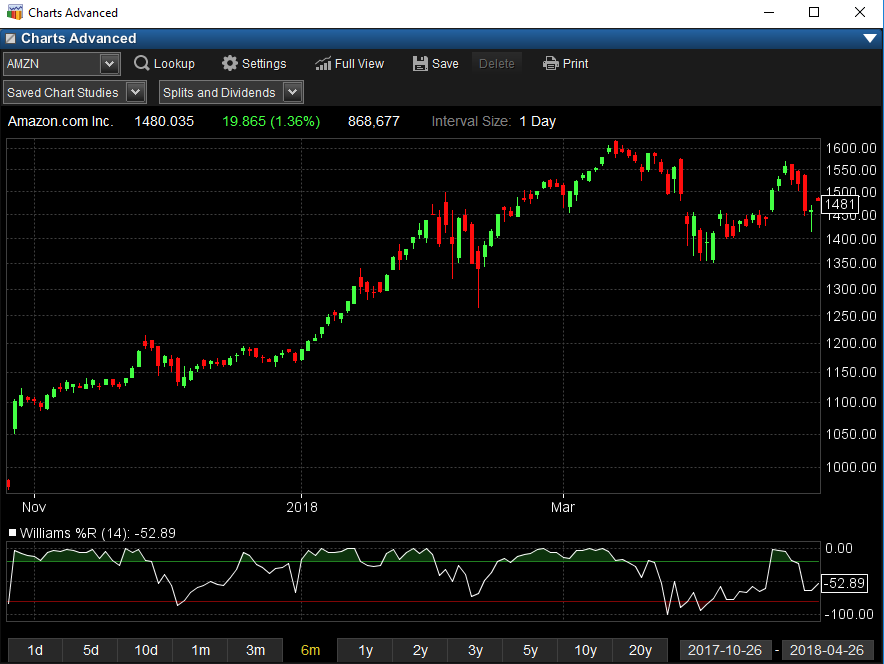Accumulation/Distribution

A momentum indicator that attempts to gauge supply and demand by determining whether investors are generally "accumulating" (buying) or "distributing" (selling) a certain stock by identifying divergences between stock price and volume flow. It is calculated using the following formula:

Acc/Dist = ((Close – Low) – (High – Close)) / (High – Low) * Period's volumeAroon Indicator

A technical indicator, developed by Tushar Chande in 1995, used for identifying trends in an underlying security and the likelihood that the trends will reverse. It is made up of two lines: one line is called "Aroon up", which measures the strength of the uptrend, and the other line is called "Aroon down", which measures the downtrend. The indicator reports the time it is taking for the price to reach, from a starting point, the highest and lowest points over a given time period, each reported as a percentage of total time. Both the Aroon up and the Aroon down fluctuate between zero and 100, with values close to 100 indicating a strong trend, and zero indicating a weak trend. The lower the Aroon up, the weaker the uptrend and the stronger the downtrend, and vice versa. The main assumption underlying this indicator is that a stock's price will close at record highs in an uptrend, and record lows in a downtrend.Aroon Oscillator

A trend-following indicator that uses aspects of the Aroon indicator ("Aroon up" and "Aroon down") to gauge the strength of a current trend and the likelihood that it will continue. The Aroon oscillator is calculated by subtracting Aroon down from Aroon up. Readings above zero indicate that an uptrend is present, while readings below zero indicate that a downtrend is present.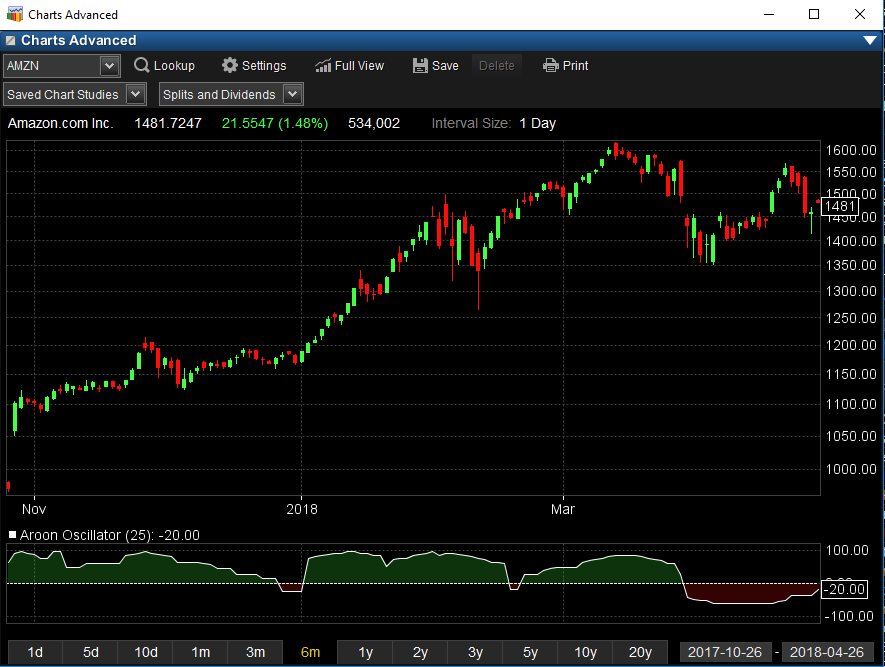Average Directional Index

The Average Directional Index (ADX), Minus Directional Indicator (-DI) and Plus Directional Indicator (+DI) represent a group of directional movement indicators that form a trading system developed by Welles Wilder.  Positive and negative directional movement form the backbone of the Directional Movement System. Wilder determined directional movement by comparing the difference between two consecutive lows with the difference between their respective highs. The Plus Directional Indicator (+DI) and Minus Directional Indicator (-DI) are derived from smoothed averages of these differences and measure trend direction over time. These two indicators are often referred to collectively as the Directional Movement Indicator (DMI). The Average Directional Index (ADX) is in turn derived from the smoothed averages of the difference between +DI and -DI, and measures the strength of the trend (regardless of direction) over time.Average True Range

A measure of volatility introduced by Welles Wilder in his book: New Concepts in Technical Trading Systems.

The True Range indicator is the greatest of the following:

-current high less the current low.

-the absolute value of the current high less the previous close.

-the absolute value of the current low less the previous close.

The Average True Range is a moving average (generally 14-days) of the True Ranges.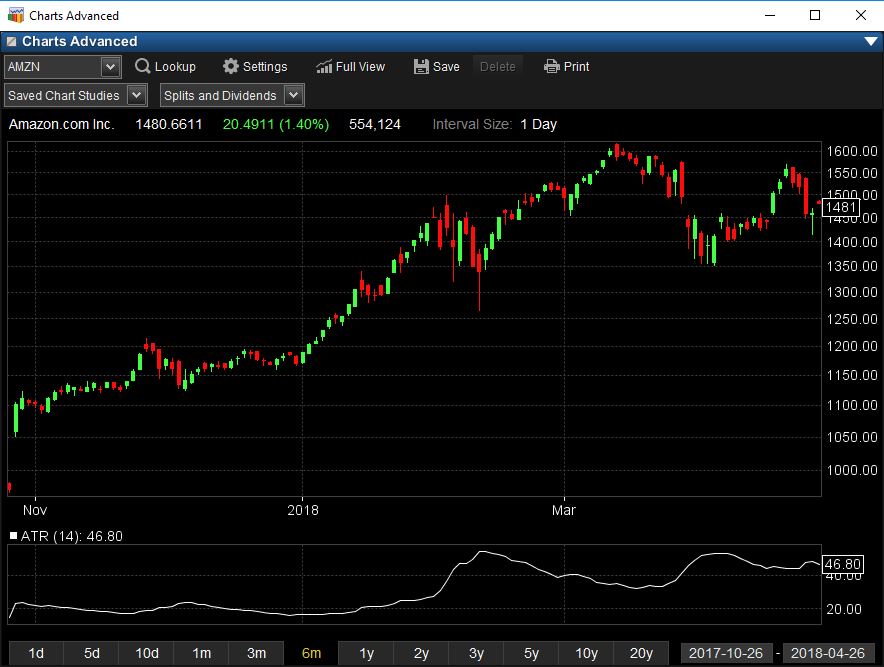Awesome Oscillator

Bill Williams’ Awesome Oscillator (AO) is designed to show current market momentum and is displayed as a histogram. The Awesome Oscillator is created using the difference between the 34-period and 5-period simple moving averages of the bar’s midpoints (H+L)/2.

Each bar of the histogram that is higher than the preceding bar is green. Each bar that is lower than the preceding bar is red.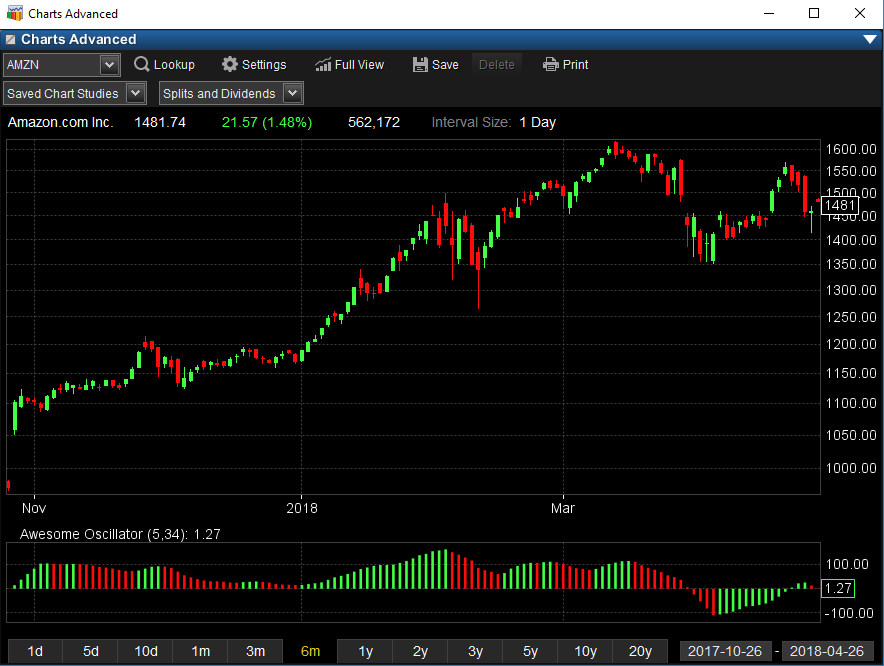Bollinger Band %B

%B quantifies a security's price relative to the upper and lower Bollinger Band.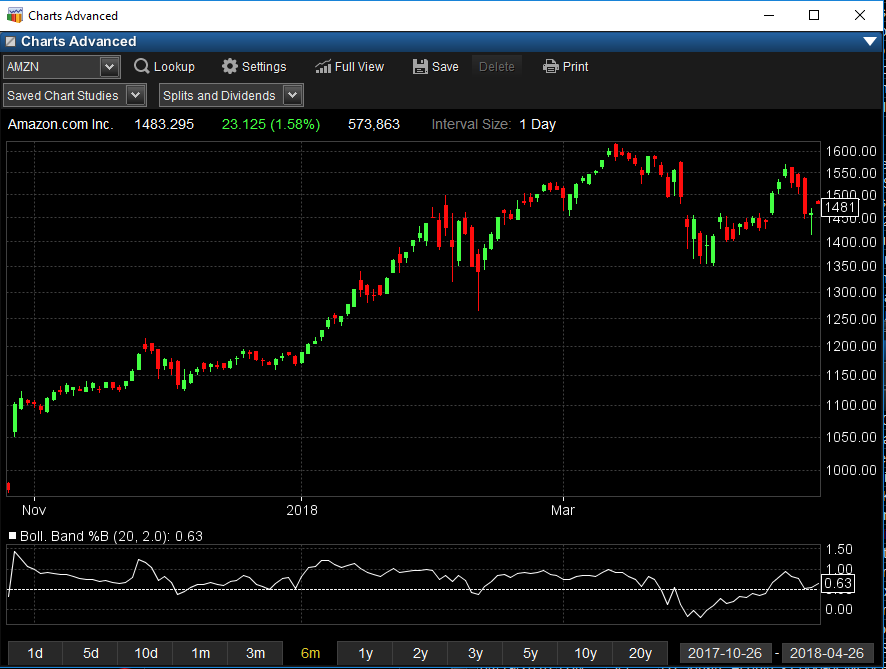Bollinger Band Width

Bollinger Band Width is an indicator derived from Bollinger Bands. In his book, Bollinger on Bollinger Bands, John Bollinger refers to Bollinger BandWidth as one of two indicators that can be derived from Bollinger Bands. The other indicator is %B.

Non-normalized BandWidth measures the distance, or difference, between the upper band and the lower band. BandWidth decreases as Bollinger Bands narrow and increases as Bollinger Bands widen. Because Bollinger Bands are based on the standard deviation, falling Bandwidth reflects decreasing volatility and rising Bandwidth reflects increasing volatility.Chaikin Money Flow

Developed by Marc Chaikin, Chaikin Money Flow measures the amount of Money Flow Volume over a specific period. Money Flow Volume forms the basis for the Accumulation Distribution Line. Instead of a cumulative total of Money Flow Volume, Chaikin Money Flow simply sums Money Flow Volume for a specific look-back period, typically 20 or 21 days. The resulting indicator fluctuates above/below the zero line just like an oscillator.Chaikin Oscillator

Developed by Marc Chaikin, the Chaikin Oscillator measures the momentum of the Accumulation Distribution Line using the MACD formula. This makes it an indicator of an indicator. The Chaikin Oscillator is the difference between the 3-day EMA of the Accumulation Distribution Line and the 10-day EMA of the Accumulation Distribution Line.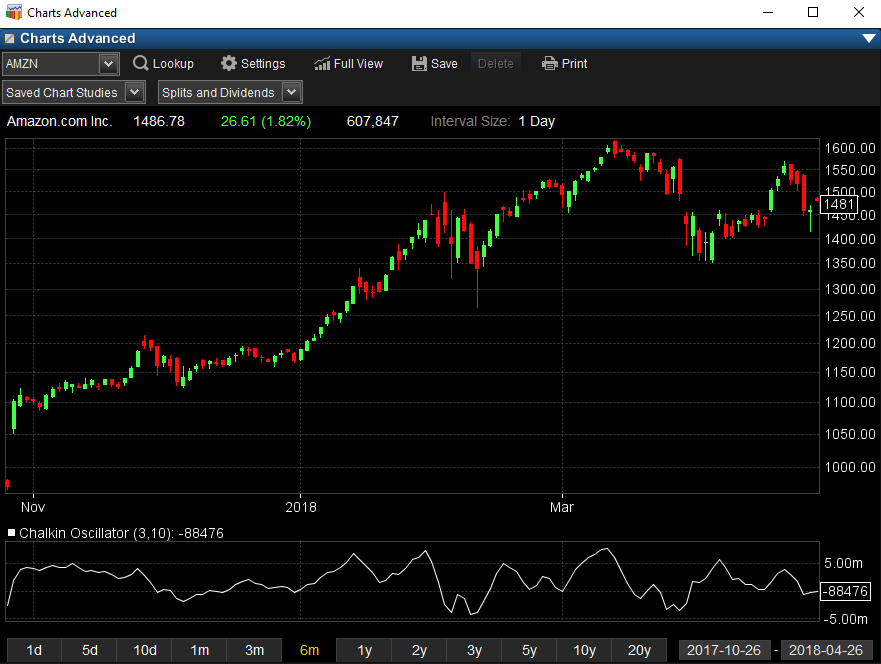Chaikin's Volatility

The Chaikin's Volatility function determines the volatility of a financial data series using the percent change in a moving average of the high versus low price over a given time.

Parameters
------------------
High             The high price of the security for each given day.
Low              The low price of the security for each given day.
MA Period    The number of bars of data to include in the moving average, including the current value.
For example, a period of 3 includes the current value and the two previous values.
A moving average period of 10 is recommended.
ROC Period  The number of bars of data to include in the percent change, not including the current value.
For example, a period of 3 compares the current value to the value three bars ago.
A rate-of-change (percent change) period of 10 is recommended.

Note that while this function is intended for use with these specific values, any values can be used for these parameters, including other price values and averaged prices.

Indicator Value
------------------------
Chaikin's Volatility indicator is calculated by taking an exponential moving average of the difference between the high and low prices over the given period of time (MA Period). A percent change (or rate-of-change) is then taken for the moving average over the given period (ROC Period). The percent rate-of-change value is traditionally multiplied by 100 for easier graphing.

Since Chaikin's Volatility uses exponential moving averages, it will have values at the beginning of the data series. However, you may want to ignore values prior to a sum of the two periods has completed.Close Location Value

A measure used in technical analysis to determine where the price of the asset closes relative to the day's high and low. The CLV ranges between +1 and -1, where a value of +1 means the close is equal to the high and a value of -1 means the close is equal to the day's low.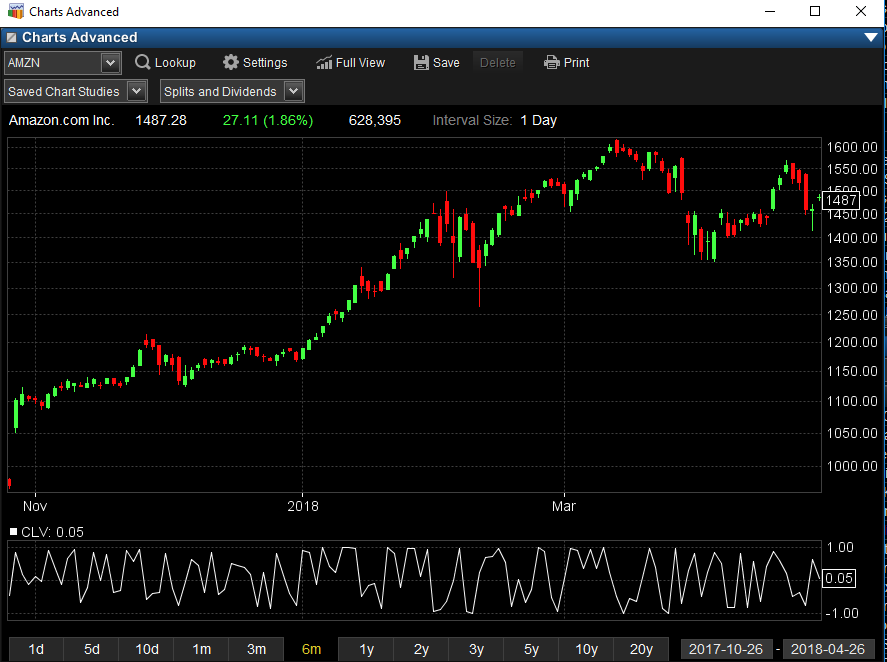Commodity Channel Index

An oscillator used in technical analysis to help determine when an investment vehicle has been overbought and oversold.  The Commodity Channel Index, first developed by Donald Lambert, quantifies the relationship between the asset's price, a moving average (MA) of the asset's price, and normal deviations (D) from that average.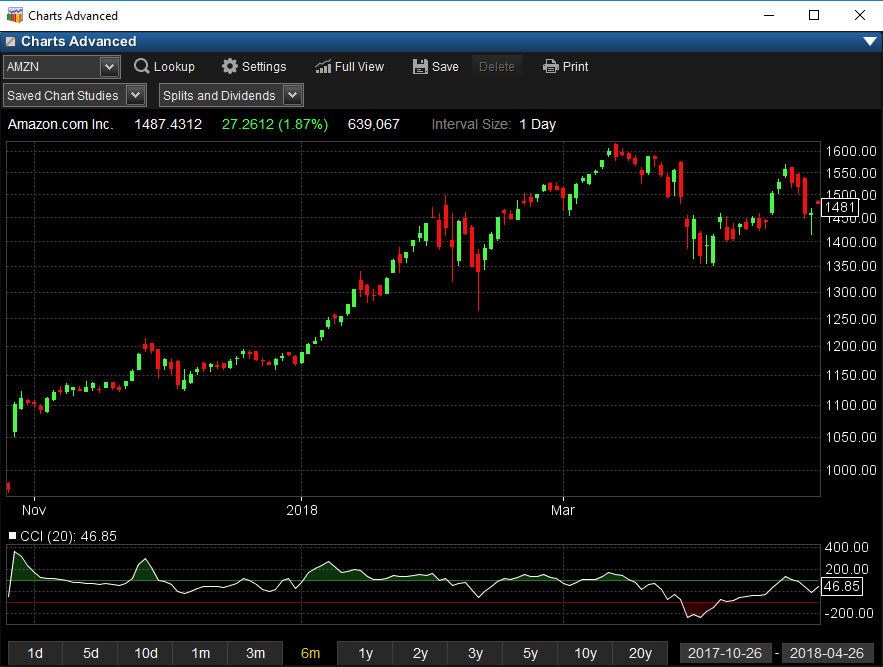Detrended Price Oscillator

The Detrended Price Oscillator ("DPO") attempts to eliminate the trend in prices. Detrended prices allow you to more easily identify cycles and overbought/oversold levels. Calculation:

To calculate the Detrended Price Oscillator, first create an n-period simple moving average (where "n" is the number of periods in the moving average).

Now, subtract the moving average "(n / 2) + 1" days ago, from the closing price. The result is the DPO.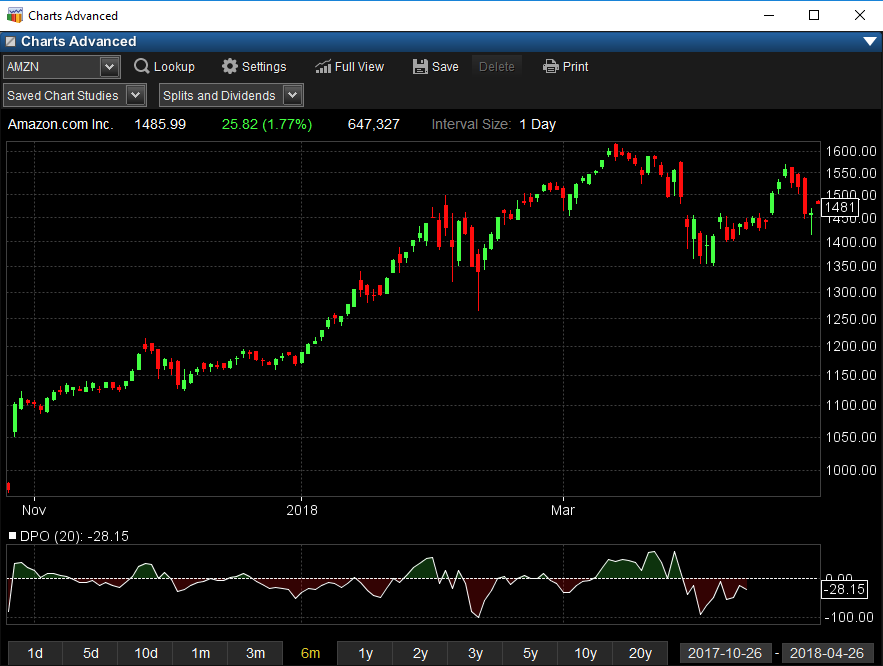Ease of Movement

A technical momentum indicator that is used to illustrate the relationship between the rate of an asset's price change and its volume. This indicator attempts to identify the amount of volume required to move prices. Generally a value greater than zero is an indication that the stock is being accumulated (bought) and negative values are used to signal increased selling pressure.

A high positive value appears when prices move upward on low volume. Strong negative numbers indicate that price is moving downward on low volume.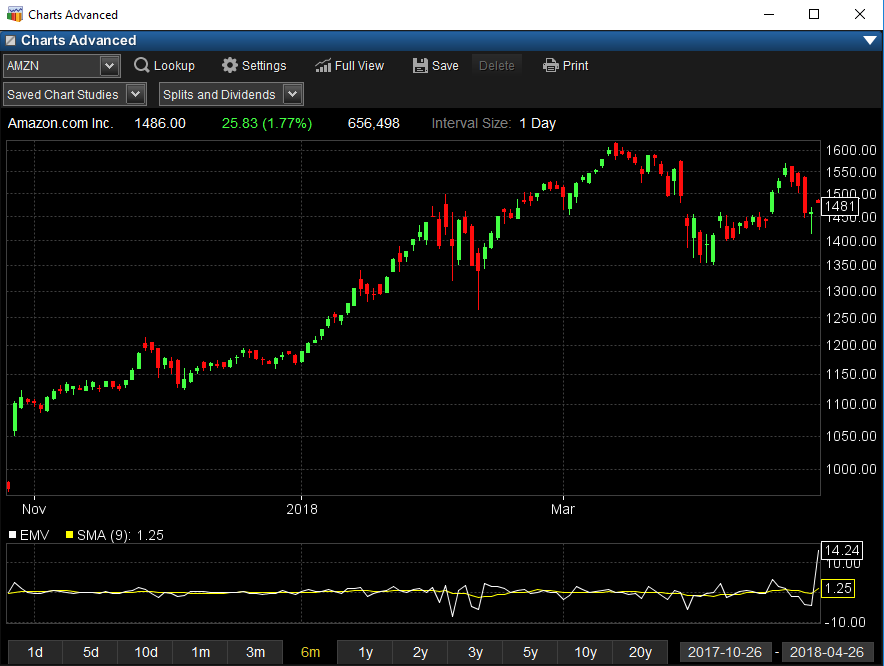Fast Stochastic

Developed by George C. Lane in the late 1950s, the Stochastic Oscillator is a momentum indicator that shows the location of the close relative to the high-low range over a set number of periods.

Fast %K = %K basic calculation

Fast %D = 3-period SMA of Fast %KInside/Outside Bars

Confirms whether it is an inside or an outside day.

Inside: A candlestick formation that occurs when the entire daily price range for a given security falls within the price range of the previous day.

Outside: Outside days are a two-bar candlestick pattern that occurs when the current day’s candle has a higher high and a lower low than the prior candle.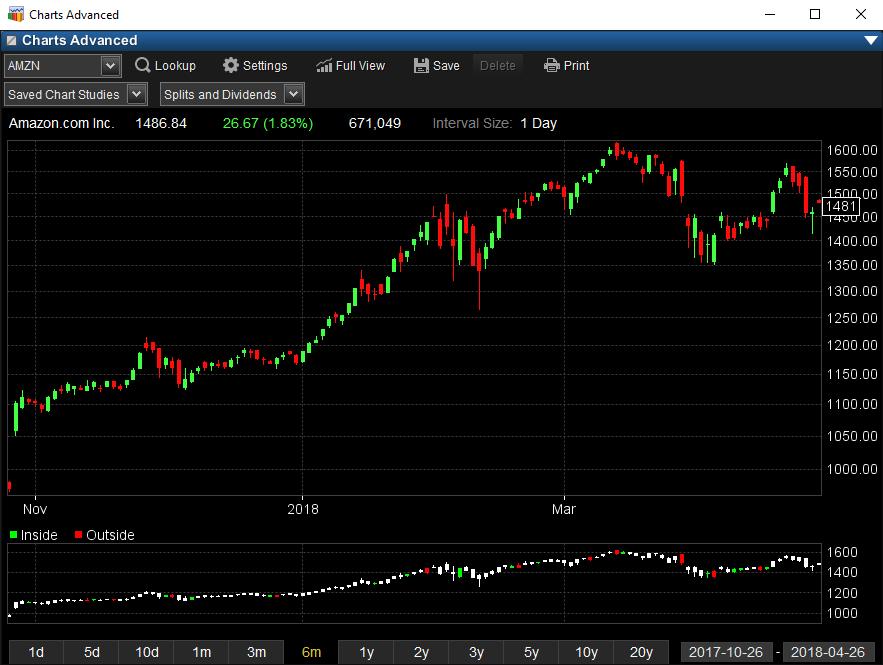Moving Average Convergence/Divergence (MACD)

A trend-following momentum indicator that shows the relationship between two moving averages of prices. The MACD is calculated by subtracting the 26-day exponential moving average (EMA) from the 12-day EMA. A nine-day EMA of the MACD, called the "signal line", is then plotted on top of the MACD, functioning as a trigger for buy and sell signals.MACD Histogram

The Histogram portion of the MACD indicator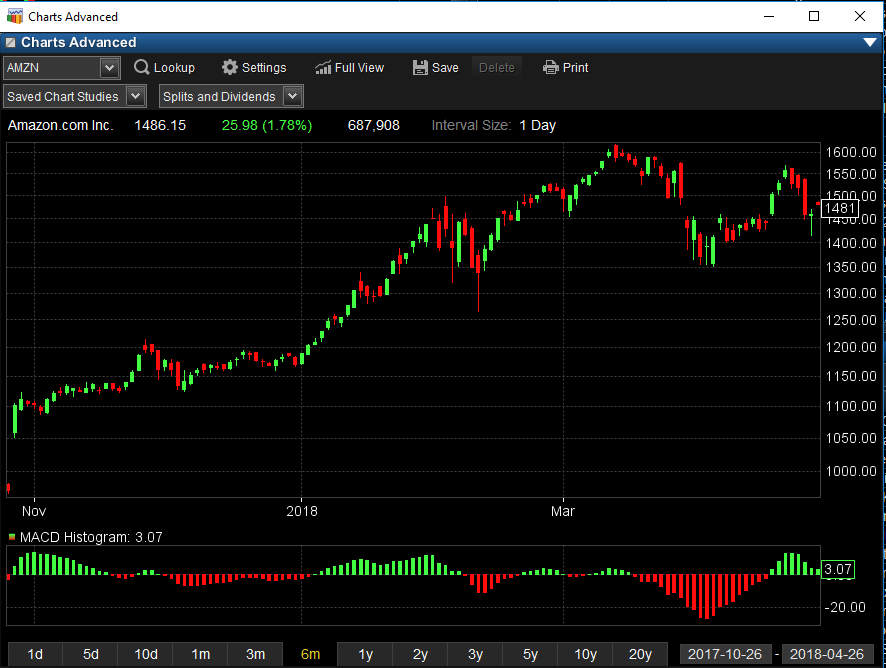Mass Index

The mass index serves for finding turns in trends. It is based on changes between maximum and minimum prices. If the amplitude gets wider, the mass index grows; if it gets narrower, the index gets smaller. The mass index was created by Donald Dorcy.

The Mass Index calculation consists of dividing the exponential moving average of the trading range for a particular bar by the exponential moving average of the exponential moving average of the trading range. The results are then added for a specific number of periods, usually 25.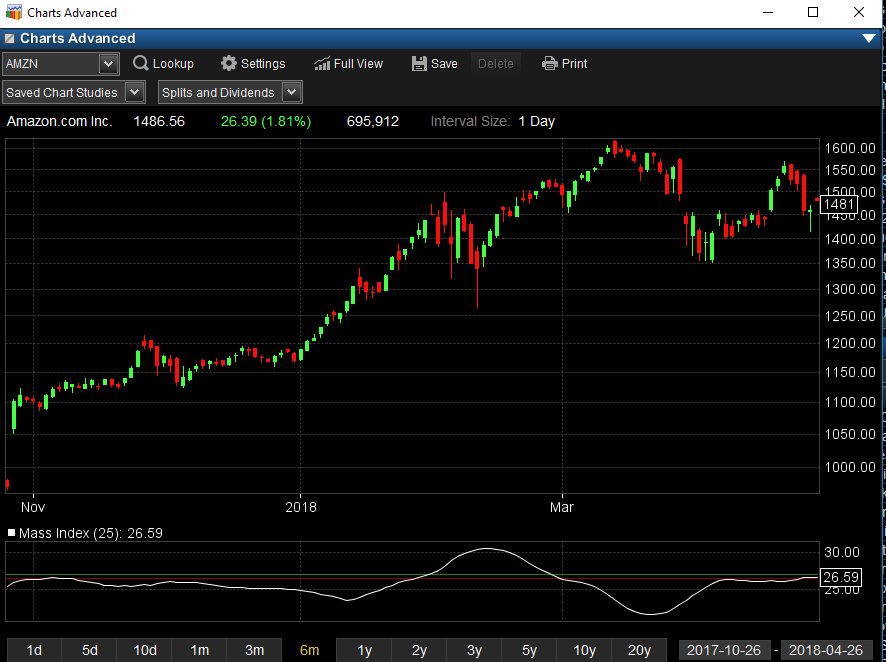Momentum

The rate of acceleration of a security's price or volume.Money Flow Index

The Money Flow Index (MFI) is an oscillator that uses both price and volume to measure buying and selling pressure. Created by Gene Quong and Avrum Soudack, MFI is also known as volume-weighted RSI. MFI starts with the typical price for each period. Money flow is positive when the typical price rises (buying pressure) and negative when the typical price declines (selling pressure). A ratio of positive and negative money flow is then plugged into an RSI formula to create an oscillator that moves between zero and one hundred.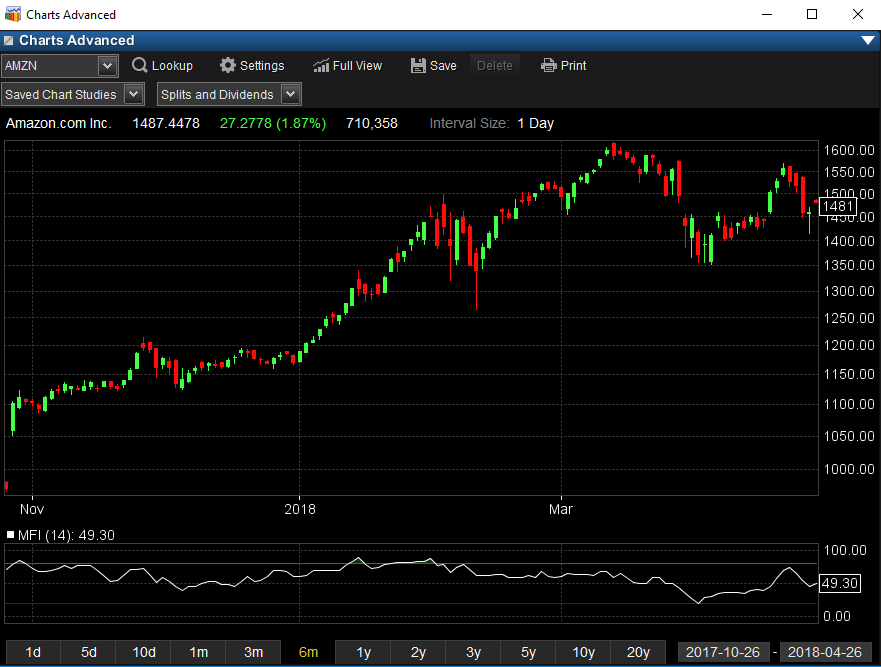Negative Volume Index

An index that focuses on days where the volume has significantly decreased from the previous day's trading.On Balance Volume

A method used in technical analysis to detect momentum, the calculation of which relates volume to price change. OBV provides a running total of volume and shows whether this volume is flowing in or out of a given security. This indicator was developed by Joe Granville.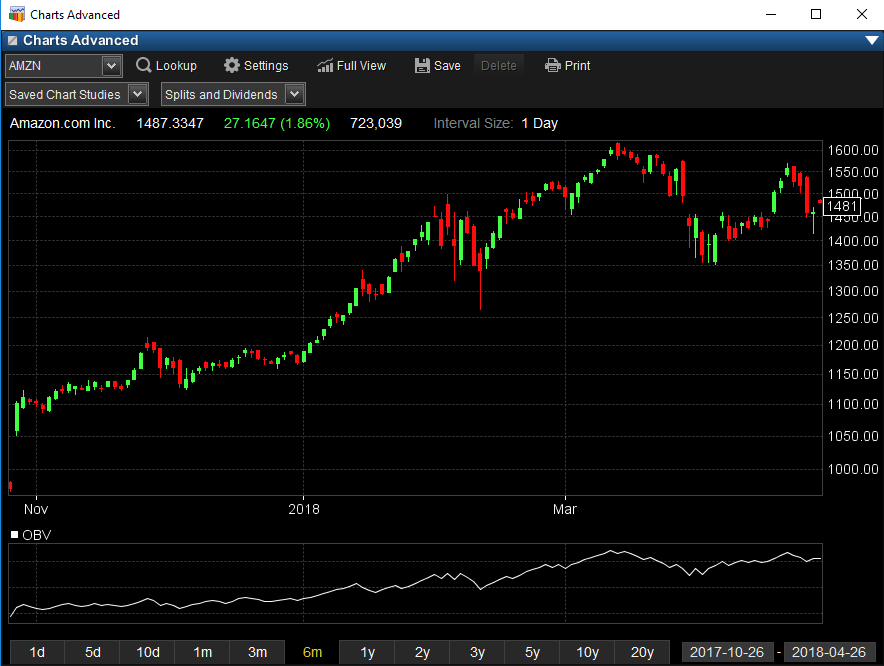Percentage Price Oscillator

A technical momentum indicator showing the relationship between two moving averages. To calculate the PPO, subtract the 26-day exponential moving average (EMA) from the nine-day EMA, and then divide this difference by the 26-day EMA. The end result is a percentage that tells the trader where the short-term average is relative to the longer-term average.

Note: We also have a Percentage Volume Oscillator available that provides the same as the above except with a focus on volume.Points Price OscillatorPoints Volume OscillatorPositive Volume Index

An index that focuses on days where the volume has significantly increased from the previous day's trading.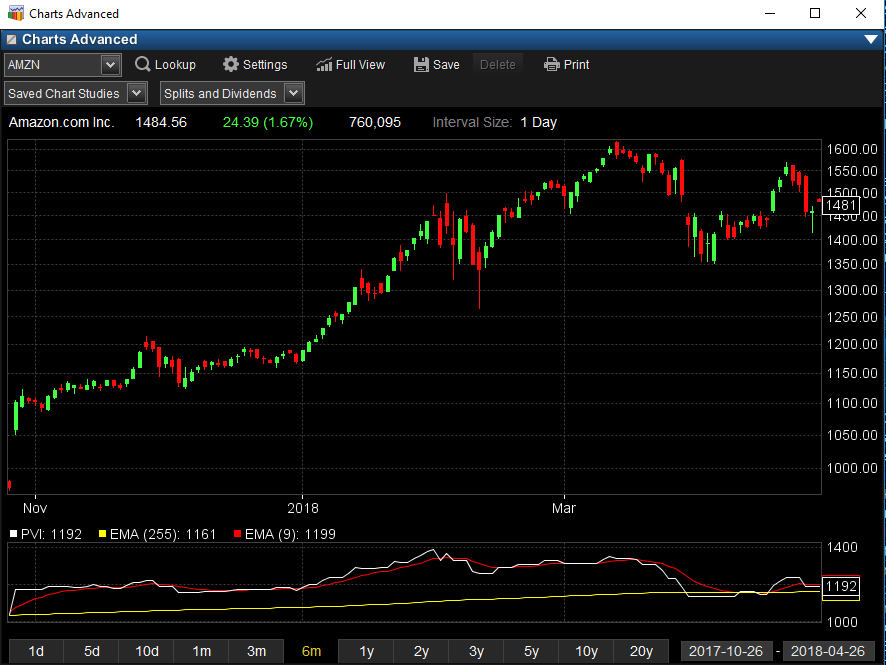Price and Volume Trend

(PVT) is a technical analysis indicator intended to relate price and volume in the stock market. PVT is based on a running total volume, with volume added according to the percentage change in closing price over the previous close.
The formula is

The starting point for the PVT total, ie. the zero point, is arbitrary. Only the shape of the resulting indicator is used, not the actual level of the total.

PVT is interpreted in similar ways to OBV. Generally the idea is that volume is higher on days with a price move in the dominant direction, for example in a strong uptrend more volume on up days than down days. So when prices are going up, PVT should be going up too, and when prices make a new rally high, PVT should too. If PVT fails to go past its previous rally high then this is a negative divergence, suggesting a weak move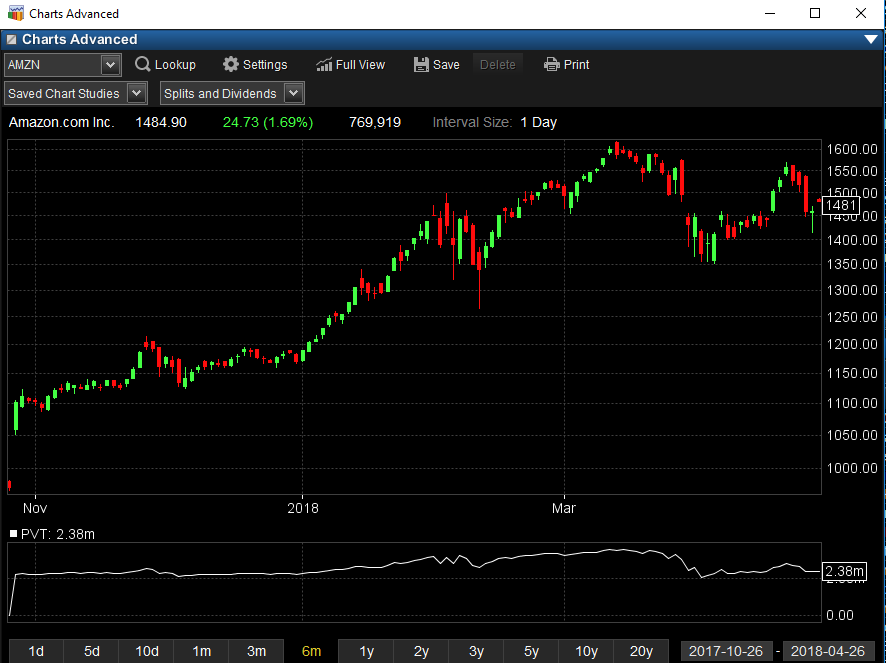Price Channel Width

Shows the distance between the upper and lower lines of a corresponding Price Channel (upper indicator). The editable parameter is used to set the moving average period on which the upper and lower bands are based.Price Rate of Change (ROC)

A technical indicator that measures the percentage change between the most recent price and the price "n" periods in the past. It is calculated by using the following formula:

(Closing Price Today - Closing Price "n" Periods Ago) / Closing Price "n" Periods Ago

ROC is classed as a price momentum indicator or a velocity indicator because it measures the rate of change or the strength of momentum of change.Relative Strength Index (RSI)

A technical momentum indicator that compares the magnitude of recent gains to recent losses in an attempt to determine overbought and oversold conditions of an asset. It is calculated using the following formula:

RSI = 100 - 100
______
1 + RS

RS = Average of x days' up closes / Average of x days' down closes

As you can see from the chart below, the RSI ranges from 0 to 100. An asset is deemed to be overbought once the RSI approaches the 70 level, meaning that it may be getting overvalued and is a good candidate for a pullback. Likewise, if the RSI approaches 30, it is an indication that the asset may be getting oversold and therefore likely to become undervalued.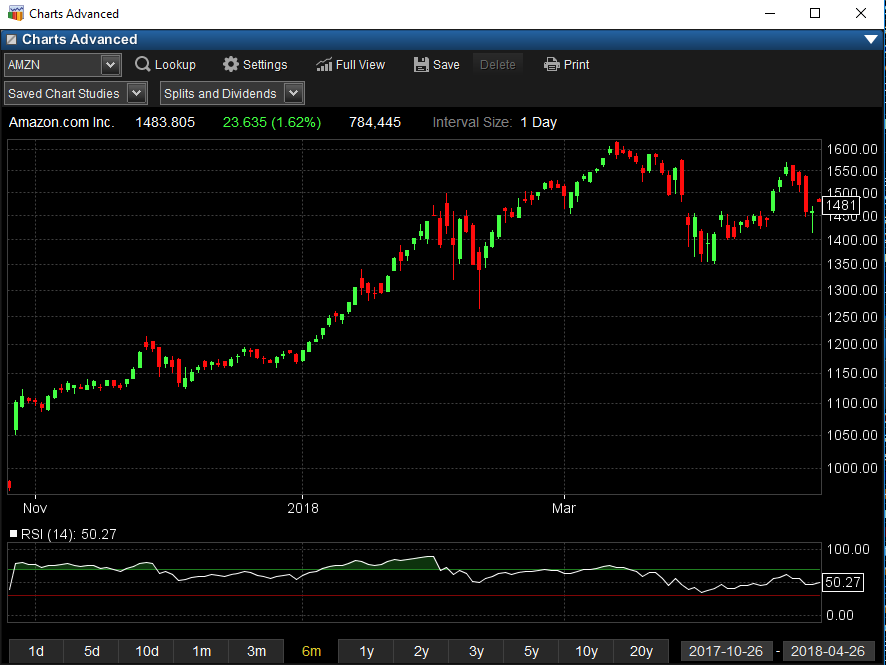Slow Stochastic

Developed by George C. Lane in the late 1950s, the Stochastic Oscillator is a momentum indicator that shows the location of the close relative to the high-low range over a set number of periods.

Slow %K = Fast %K smoothed with 3-period SMA
Slow %D = 3-period SMA of Slow %K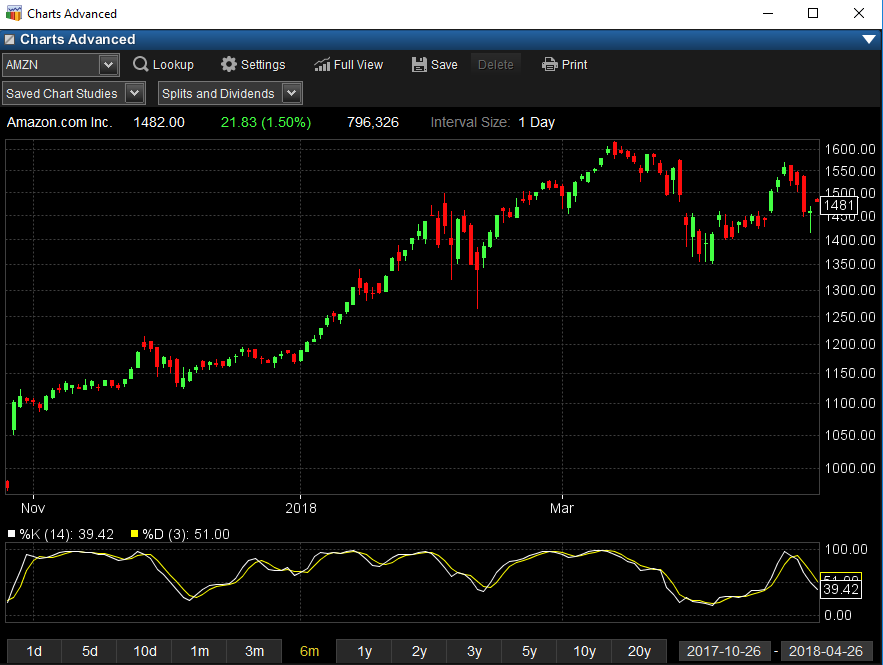Standard Deviation

1. A measure of the dispersion of a set of data from its mean. The more spread apart the data, the higher the deviation. Standard deviation is calculated as the square root of variance.

2. In finance, standard deviation is applied to the annual rate of return of an investment to measure the investment's volatility. Standard deviation is also known as historical volatility and is used by investors as a gauge for the amount of expected volatility.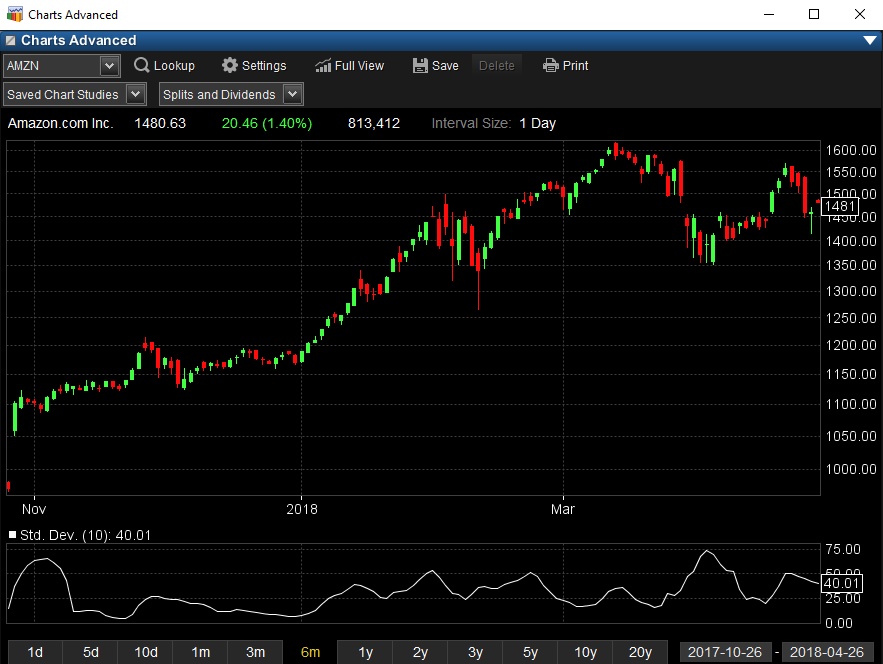Stochastic RSI

Introduced by Tuschar Chande and Stanley Kroll in their December, 1992 Stocks and Commodities article entitled, “Stochastic RSI and Dynamic Momentum Index”, the Stochastic RSI Oscillator attempts to combine ideas from two indicators - the Relative Strength Index (RSI) and the Stochastic Oscillator. As its name implies, the Stochastic RSI Oscillator is the RSI run through the stochastic algorithm. The Stochastic RSI Oscillator is a momentum indicator designed to show the relation of the current RSI value relative to its high/low range over a given number of periods using a scale of 0-100.

The Stochastic RSI Oscillator, much like the other stochastic oscillators, is typically plotted as 2 lines: the StochRSI line and %D. The StochRSI is the main (fast) line and %D is the signal (slow) line.

The Stochastic RSI Oscillator is calculated by the formula:

StochRSI = ((Today's RSI - Lowest RSI Low in %K Periods) / (Highest RSI High in %K Periods - Lowest RSI Low in %K Periods)) * 100

Slowing StochRSI = 3-period moving average of StochRSI; using a 1-period moving average of StochRSI eliminates this slowing (or smoothing) of the StochRSI

%D = 3-period moving average of Slowing StochRSI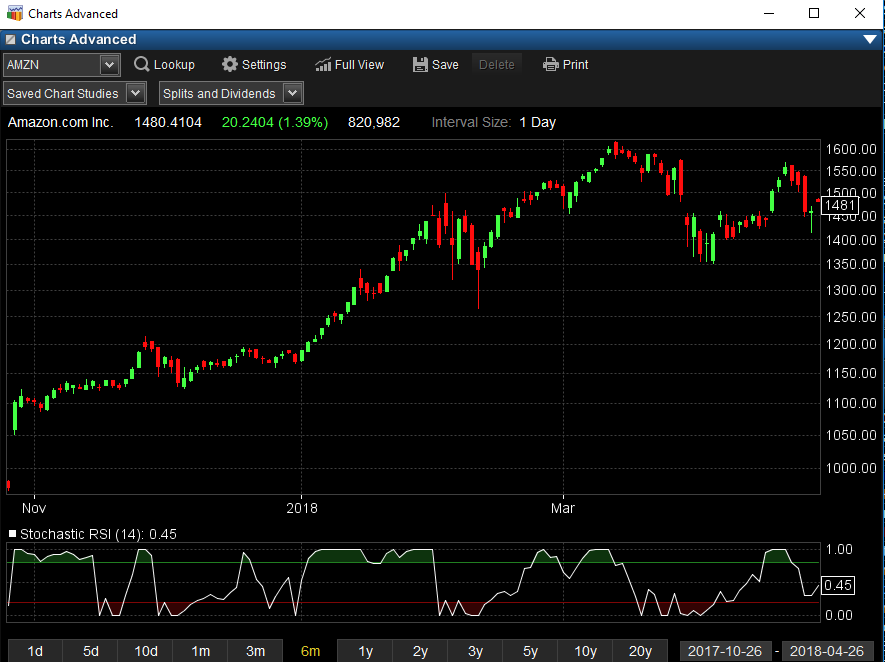TRIX

TRIX is a momentum indicator that displays the percent rate-of-change of a triple exponentially smoothed moving average of a security's closing price. It was developed in the early 1980's by Jack Hutson, an editor for Technical Analysis of Stocks and Commodities magazine. Oscillating around a zero line, TRIX is designed to filter out stock movements that are insignificant to the larger trend of the stock. The user selects a number of periods (such as 15) with which to create the moving average, and those cycles that are shorter than that period are filtered out.

The TRIX is a leading indicator and can be used to anticipate turning points in a trend through its divergence with the security price. Likewise, it is common to plot a moving average with a smaller period (such as 9) and use it as a "signal line" to anticipate where the TRIX is heading. TRIX line crossovers with its "signal line" can be used as buy/sell signals as well.

Calculation

To calculate TRIX, you must first pick a period with which to create an exponential moving average of the closing prices. For a 15-day period:

1. Calculate the 15-day exponential moving average of the closing price.

2. Calculate the 15-day exponential moving average of the moving average calculated in step #1.

3. Calculate the 15-day exponential moving average of the moving average calculated in step #2. You now have triple exponentially smoothed the moving average of closing prices, greatly reducing volatility.

4. Finally, calculate the 1-day percent change of the moving average calculated in step #3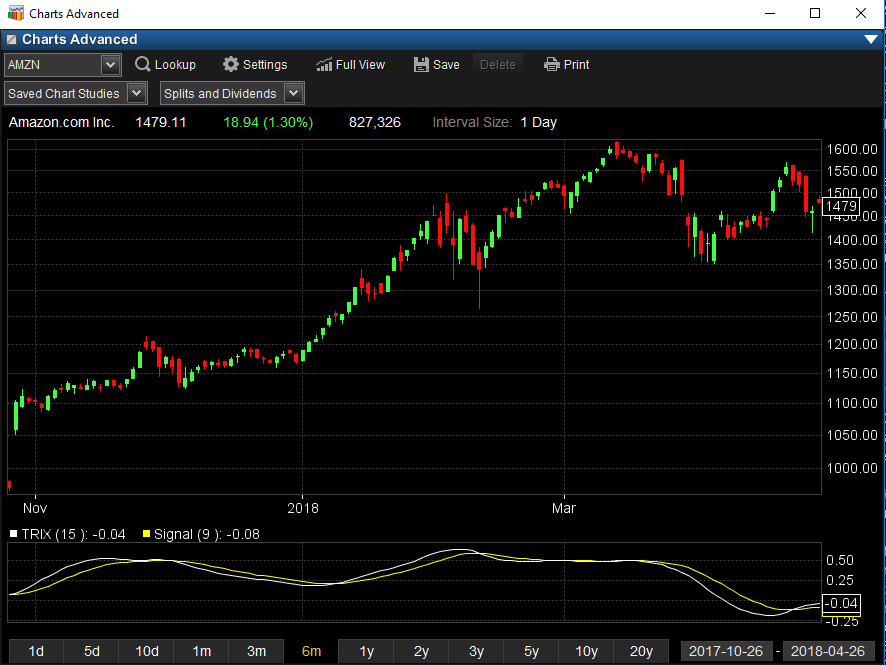True RangeUltimate Oscillator

A technical indicator invented by Larry Williams that uses the weighted average of three different time periods to reduce the volatility and false transaction signals that are associated with many other indicators that mainly rely on a single time period.Vertical Horizontal Filer

Vertical Horizontal Filter (VHF) is a trending and ranging indicator authored by Adam White. The VHF uses the highest close minus the lowest close divided by the sum of the absolute value of the difference of the highest and lowest over a user defined time period.Volume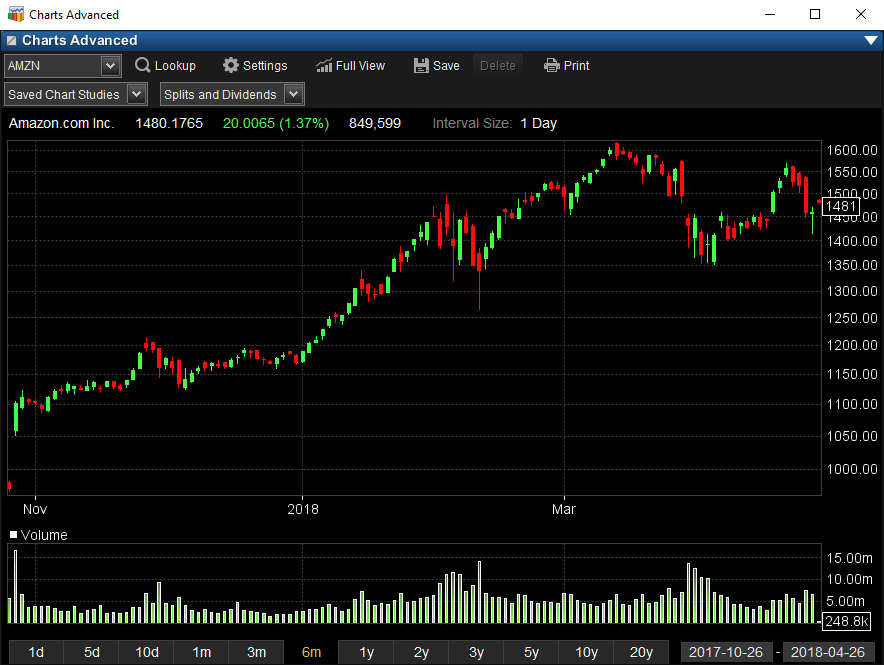Volume+

Volume plus an adjustable moving average.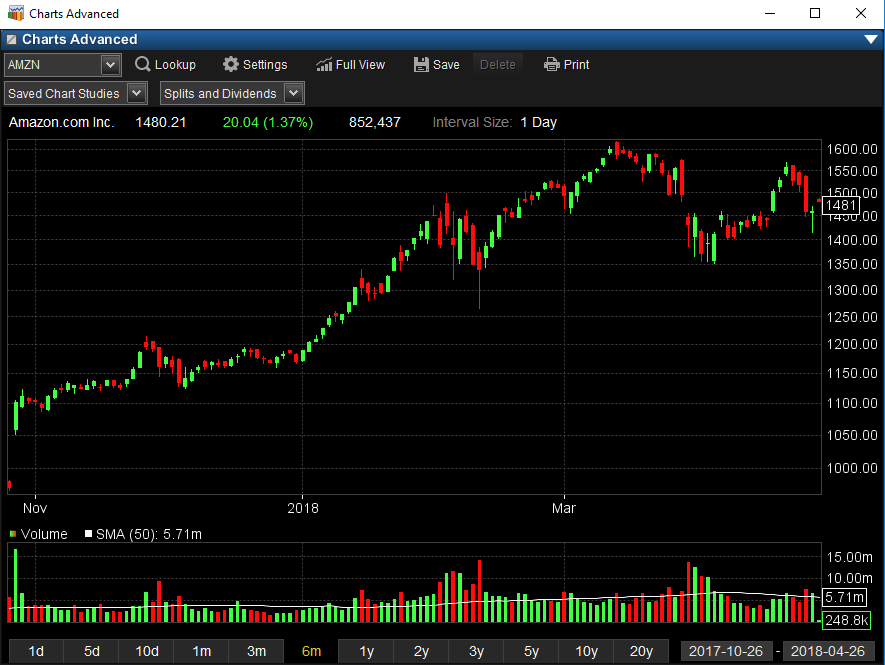Volume Rate of Change (ROC)

The volume rate of change is the indicator that shows whether or not a volume trend is developing in either an up or down direction. You may be familiar with price rate of change (discussed here), which shows an investor the rate of change measured by the issue's closing price. To calculate you need to divide the volume change over the last n-periods (days, weeks or months) by the volume n-periods ago. The answer is a percentage change of the volume over the last n-periods. Now, what does this mean? If the volume today is higher than n-days (or weeks or months) ago, the rate of change will be a plus number. If the volume is lower, the ROC will be minus number. This allows us to look at the speed at which the volume is changing.Williams %R

In technical analysis, this is a momentum indicator measuring overbought and oversold levels, similar to a stochastic oscillator. It was developed by Larry Williams and compares a stock's close to the high-low range over a certain period of time, usually 14 days.